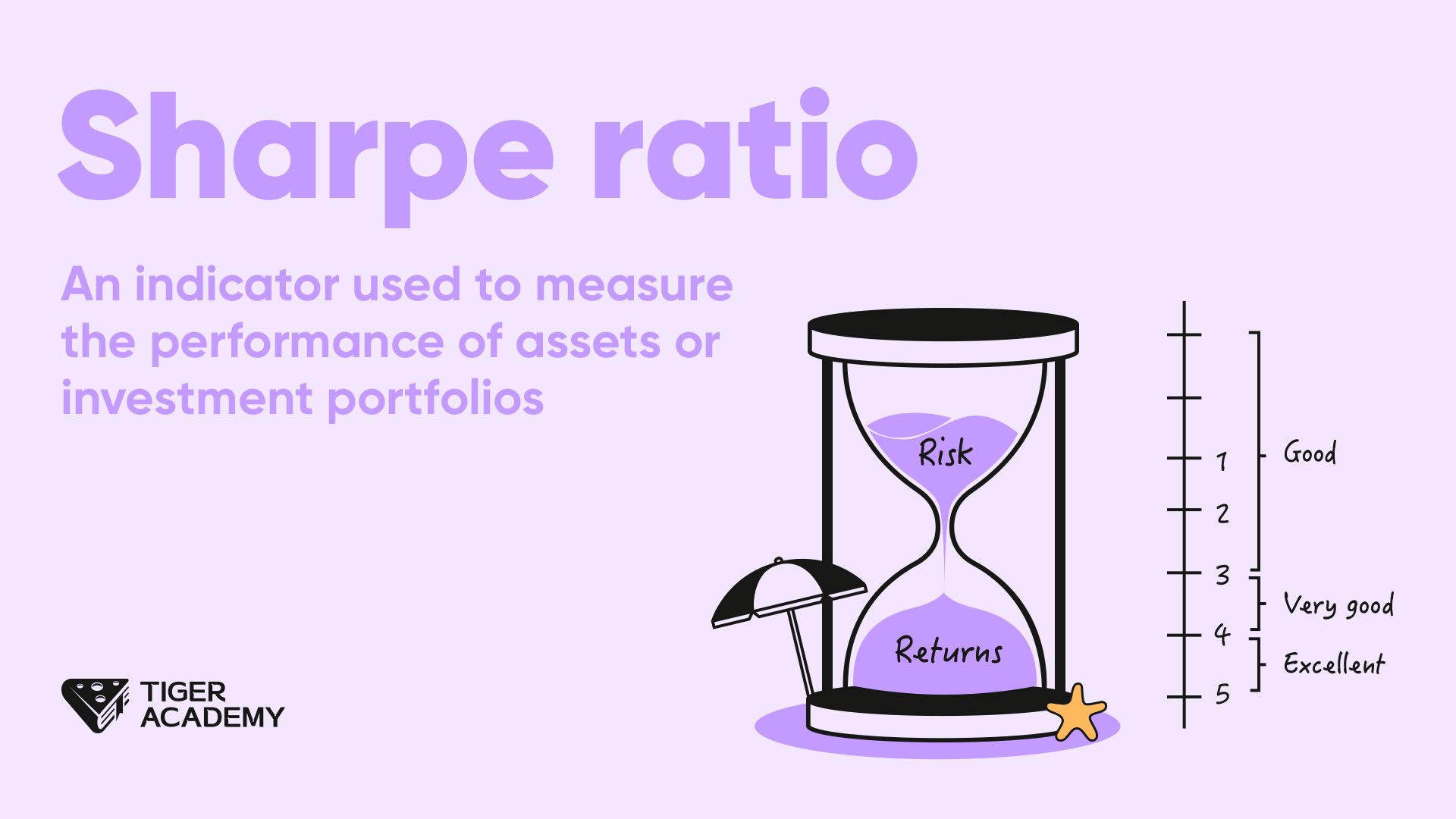## Sharpe ratio

08 AugThe Sharpe Ratio is a financial metric that evaluates the effectiveness of an investment by comparing the risk-adjusted return (excess return relative to risk-free assets) of a portfolio or asset with its volatility (i.e., risk).

The Sharpe Ratio helps investors assess the risk-adjusted performance of a portfolio or asset and aids them in making decisions among different investment choices.

In simple terms, the Sharpe Ratio is a measure of the performance of an asset or investment portfolio.

The formula to calculate the Sharpe Ratio is: Sharpe Ratio = (Rp - Rf) / σp.

Here, Rp represents the expected return of the portfolio or asset, Rf is the return of a risk-free asset, and σp is the standard deviation of the portfolio or asset, which measures its volatility or risk.

A higher Sharpe Ratio indicates higher excess returns per unit of risk and implies better performance of the portfolio. Conversely, a lower Sharpe Ratio suggests poorer performance of the portfolio.

Let's take an example:

Suppose you are considering two investment portfolios, A and B, with Sharpe Ratios of 0.8 and 1.2, respectively. Based on the comparison of the Sharpe Ratios, portfolio B has better risk-adjusted returns as its Sharpe Ratio is higher, indicating higher excess returns per unit of risk.

Regarding this metric, it is important to note the following:

The Sharpe Ratio as a performance evaluation metric considers only excess returns and volatility, without taking into account other risk factors such as market risk or liquidity risk. Therefore, when using the Sharpe Ratio, you should consider other factors in conjunction and align them with your investment goals and risk tolerance.

Here's another example:

For instance, in an investment decision, you might compare two investment portfolios, portfolio A and portfolio B, with Sharpe Ratios of 0.8 and 1.2, respectively.

Although the Sharpe Ratio indicates that portfolio B has better risk-adjusted performance, you should also consider other factors such as investment objectives, asset allocation, and portfolio composition of both portfolio A and portfolio B.

If your investment goal is long-term capital appreciation, and portfolio A's asset allocation aligns better with your investment objectives and risk preferences, you might still choose portfolio A even if its Sharpe Ratio is lower.